# How to Calculate a Percentage of a Number in Excel?

• Last Updated : 24 Jun, 2022

Excel is a tool for storing and managing a large volume of data. It uses rows and columns to store the data. This tool is used for the analysis of data to get insights from it. Generally, this is used by the financial professional for accounting purposes but it can be used by anyone. Data analysis can be performed in many ways like visual analysis using graphs, mathematical or trend analysis using formulas, etc. Let’s learn how can we calculate a percentage of numbers in excel. The percentage means, the proportion per hundred (out of 100). It is represented with the symbol ‘%’.

Percentage = (Numerator/Denominator) × 100

### Percentage of numbers using excel

For example, suppose we want to calculate 20% of 200. Type the following formula into an Excel cell,

= 20% * 200

Example 1: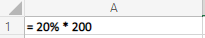Output: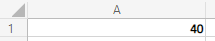We can take reference of other cell values also, instead of using direct numerical values. Suppose we have a cell containing the salary of an employee and we want to calculate pf contribution by him, that is 12% of his salary.

Example 2: A2 cell contains salary and we want the pf contribution amount in B2 cell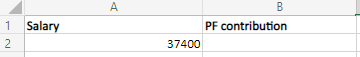Solution:

Use cell name in place of number whose percentage is required.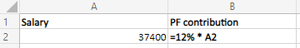Output: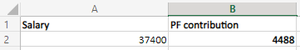My Personal Notes arrow_drop_up
Recommended Articles
Page :